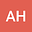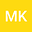On the derivations‎, ‎generalized derivations and ternary derivations of degree $n$
••• Amin Hosseini,
Amin Hosseini
Kashmar Higher Education Institute, Kashmar, Iran

Corresponding Author:[email protected]

Author Profile‎In this paper‎, ‎we introduce the concepts of derivation of degree $n$‎, ‎generalized derivation of degree $n$ and ternary derivation of degree $n$‎, ‎where $n$ is a positive integer‎, ‎and then we study algebraic properties of these mappings‎. ‎For instance‎, ‎we study the image of derivations of degree $n$ on algebras and in this regard we prove that‎, ‎under certain conditions‎, ‎every derivation of degree $n$ on an algebra maps the algebra into its Jacobson radical‎. ‎Also‎, ‎we present some characterizations of these mappings on algebras‎. ‎For example‎, ‎under certain assumptions‎, ‎we show that if $f$ is an additive generalized derivation of degree $n$ with an associated mapping $d$‎, ‎then either $f$ is a linear generalized derivation with the associated linear derivation $d$ or $f$ and $d$ are identically zero‎. ‎Some other related results are also established‎.